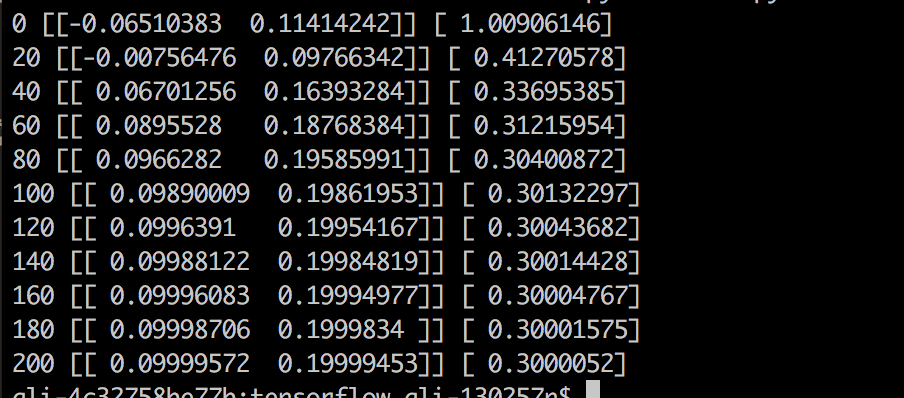Tensorflow 是一个使用数据流图进行数值计算的开源软件库。它由谷歌公司开源出去，方便全世界的开发者使用它来进行机器学习(Machine Learning)

TensorFlow是谷歌基于DistBelief进行研发的第二代人工智能学习系统，其命名来源于本身的运行原理。Tensor（张量）意味着N维数组，Flow（流）意味着基于数据流图的计算，TensorFlow为张量从图象的一端流动到另一端计算过程。TensorFlow是将复杂的数据结构传输至人工智能神经网中进行分析和处理过程的系统。
TensorFlow可被用于语音识别或图像识别等多项机器深度学习领域，对2011年开发的深度学习基础架构DistBelief进行了各方面的改进，它可在小到一部智能手机、大到数千台数据中心服务器的各种设备上运行。TensorFlow将完全开源，任何人都可以用(百度百科)

### 安装

``````sudo easy_install pip

``````

``````RuntimeError: module compiled against API version 0xa but this version of numpy is 0x9Traceback (most recent call last):File ““, line 1, in
``````

``````sudo easy_install --upgrade numpy
``````

Tensorflow 可以支持诸如 C++ , Java 等语言来运行，但是我们还是推荐你使用 Python 来学习，因为它对语言基础的要求并不是那么高，熟悉基本的语法即可。Python 基础

``````import tensorflow as tf
import numpy as np

# 使用 NumPy 生成假数据(phony data), 总共 100 个点.
x_data = np.float32(np.random.rand(2, 100)) # 随机输入
y_data = np.dot([0.100, 0.200], x_data) + 0.300

# 构造一个线性模型
#
b = tf.Variable(tf.zeros())
W = tf.Variable(tf.random_uniform([1, 2], -1.0, 1.0))
y = tf.matmul(W, x_data) + b

# 最小化方差
loss = tf.reduce_mean(tf.square(y - y_data))
train = optimizer.minimize(loss)

# 初始化变量
init = tf.initialize_all_variables()

# 启动图 (graph)
sess = tf.Session()
sess.run(init)

# 拟合平面
for step in xrange(0, 201):
sess.run(train)
if step % 20 == 0:
print step, sess.run(W), sess.run(b)

# 得到最佳拟合结果 W: [[0.100  0.200]], b: [0.300]
``````

``````python your_script.py
``````

``````SyntaxError: Non-ASCII character '\xe4' in file test.py on line 2, but no encoding declared; see http://www.python.org/peps/pep-0263.html for details
``````

`````` # -*- coding: UTF-8 -*- 或者 #coding=utf-8 就行了
``````You Can Speak "Hi" to Me in Those Ways